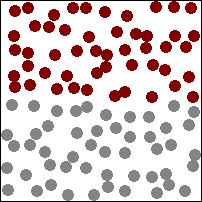## Sunday, 16 June 2013

### Mosh Pits and Molecules

What do mosh pits and molecules have in common? Apparently their velocities can be described by the same statistics. For those born before heavy metal music, moshing is a style of dance often performed at the front of a stage at a live concert. People will energetically slam and push into each other.

### Molecules' motionIdeal gas animation (http://commons.wikimedia.org/wiki/File:Gaz_molecules.gif)
If I had been moshing and were to lift my arm up afterwards, others in the room would notice my body odour from the molecules in my armpit diffusing into the room. This random motion can be modeled using the Boltzmann distribution of energies:
$\frac{N_{i}}{N}=\frac{g_{i}exp(-E_{i}/kT)}{\sum_{j}g_{j}exp(-E_{j}/kT)}$
By using this formula we can think of all the different ways the molecules could be in (microstate) and we can work out the probability, which is in a particular arrangement. We can work out the distribution of the momentum (vector). $E=p^{2}/2m$. By putting this into the Maxwell distribution we can calculate the distribution of the velocity (vector) in a single direction.
$f_{v}(v_{i})=\sqrt{\frac{m}{2\pi kT}}exp(\frac{-mv_{i}^{2}}{2kT})$
Working out the distribution of speed which is now in three dimensions is easiest done using polar coordinates (like that of a world map) to switch from x,y,z to $v,\theta,\phi$ This requires a coordinate transformation.
$dv_{x}d_{y}d_{z}=v^{2}sin(\phi)dv d\theta d\phi$
This yields the probability distribution in three dimensions.
$f(v)=\sqrt{(\frac{m}{2 \pi kT})^{3}}4 \pi v^{2}exp(-\frac{mv^{2}}{2kT})$
The squared speed term says that the probability of having zero speed is zero and increases. The long tail comes from the exponential term. The assumptions made in modeling molecules like this are that:
• The molecule has no volume.
• All collisions are elastic (energy is conserved).
• The molecules move randomly and are distinguishable from each other.

### Moshing motion

Particle tracking was applied to people in mosh and circle pits in heavy metal concerts. The most remarkable finding was that the velocities of the people in the mosh pit matched a Maxwell-Boltzmann distribution. The velocity-velocity correlation was then found to decay away with a decay length of 0.39+-0.03 m (about shoulder width apart) which suggests that the people move distinctly from each other.

This motion is then modelled using flocking based forces, repulsion forces, propulsion forces and some noise. This is found to model the mosh pit accurately.

They then compare three different time scales - flocking time scales, noise time and collision time-related to the mean free path. Random motion from collisions and noise over long time scales reproduce statistics of classical gases which is how the non-equilibrium system can show equilibrium characteristics. When flocking dominates then you can get vortex states which result in angular momentum. These are called circle pits. Interestingly, the majority of these observed rotate counter-clockwise (95%). This was not predicted by the model but may be due to handedness/footedness. This presents an interesting analogue to a classical gas with all the particles distinguishable not correlated with enough chaos to show Maxwell-Boltzmann distribution in the macroscopic world. I wonder if Boltzmann would have been into heavy metal moshing. Maybe it’s just the beard.

They also have this awesome javascript mosh pit simulator.

Silverberg, J. L., Bierbaum, M., Sethna, J. P., & Cohen, I. (2013). Collective Motion of Humans in Mosh and Circle Pits at Heavy Metal Concerts. Physical Review Letters, 110(22), 228701. doi:10.1103/PhysRevLett.110.228701

## Tuesday, 4 June 2013

### TrA Data collection

Explaining how we collect data with our transient absorption spectrometer is often very difficult.

Many people in a chemistry lab know how to collect a UV/Vis spectrum of a ground state molecule's absorbance of light at different wavelengths. However, transient absorption spectroscopy (TrA) looks at the excited molecule's spectrum and how it changes in time after its initial excitation.

These three-dimensional graphs contains so much more information than a typical absorption measurement. The transition energies are shown in the spectrum and how these change in time can be related to the kinetics. Together, these are what makes TrA so powerful - the ability to understand how the energetics of excited molecules change with time.

In the lab we collect the data by collecting the spectrum of the excited molecule using a probe pulse of white light (we also have to subtract the response without the pump). We can delay the probe relative to the pump and collect a spectrum at a later time. This is how we then build up the 3D surface.

The animation shows a TrA spectrum (which I made up). I masked the data, saved a picture and then used ImageJ to make the GIF. I will upload the code after my exams.

## Sunday, 2 June 2013

### PyTrA v.1.0

The first version of PyTrA is now released and available on the Photon Factory webpage for download.

The new version has a new interface that makes data exploration much easier. Fitting can be done of the kinetics and the spectra and Markov Chain Monte Carlo can be applied to both dimensional fits.

This allows for model checking using Bayesian methods that were not possible before. The noise of the data is also included in the MCMC fitting this allows for quantification of noise levels and to determine the signal to noise ratio accurately.

Next version will add variable projection global fitting as well as MCMC global fitting coupled with custom models. This should allow complicated models including multiple decays and complex kinetic models as well as artifacts and bleaches to be fitted using Bayesian or global methods.